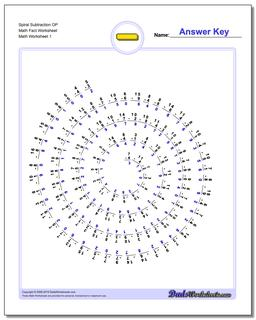# Math Worksheets: Subtraction: Subtraction: Spiral Subtraction OP Math Fact Worksheet## Spiral Subtraction OP Math Fact Worksheet

PropertyValue
DescriptionSpiral Subtraction OP Math Fact Worksheet: Tired of the same old math fact worksheets with rows and rows of problems? Time to try something unique. These worksheets present the subtraction facts in a spiral layout that provides fun a twist on memorizing the basic math facts. They use the same fact layouts as the spaceship math sheets above, so try the first two sets worksheets if you are looking for the full set of subtraction facts or practice without the easier problems, or look at the others for an incremental approach to learning everything related to the trusty minus sign!
Resource TypeWorksheet